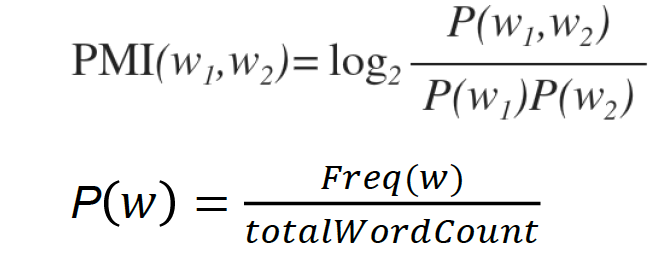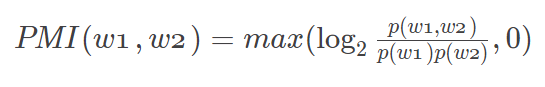# Pointwise mutual information (PMI) in NLP

Natural Language Processing (NLP) has secured so much acceptance recently as there are many live projects running and now it's not just limited to academics only. Use cases of NLP can be seen across industries like understanding customers' issues, predicting the next word user is planning to type in the keyboard, automatic text summarization etc. Many researchers across the world trained NLP models in several human languages like English, Spanish, French, Mandarin etc so that benefit of NLP can be seen in every society. In this post we will talk about one of the most useful NLP metric called Pointwise mutual information (PMI) to identify words that can go together along with its implementation in Python and R.

## What is Pointwise mutual information?

PMI helps us to find related words. In other words, it explains how likely the co-occurrence of two words than we would expect by chance. For example the word "Data Science" has a specific meaning when these two words "Data" and "Science" go together. Otherwise meaning of these two words are independent. Similarly "Great Britain" is meaningful since we know the word "Great" can be used with several other words but not so relevant in meaning like "Great UK, Great London, Great Dubai etc."

When words 'w1' and 'w2' are independent, their joint probability is equal to the product of their individual probabilities. Imagine when the formula of PMI as shown below returns 0, it means the numerator and denominator is same and then taking log of 1 produces 0. In simple words it means the words together has NO specific meaning or relevance. Question arises what are we trying to achieve here. We are focusing on the words which have high joint probability with the other word but having not so high probability of occurrence if words are considered separately. It implies that this word pair has a specific meaning.Our objective is to find pairs of words that have high pointwise mutual information.

## Steps to compute PMI

Let's understand with an example. Suppose you have the following text and you are asked to calculate PMI scores based on that.
`this is a foo bar bar black sheep  foo bar bar black sheep foo bar bar black sheep shep bar bar black sentence`
Step 1: Convert it to tokens

There are 23 tokens (words) in the text. Tokens are shown below.

```  "this"  "is"    "a"     "foo"   "bar"   "bar"   "black" "sheep"
 "foo"   "bar"   "bar"   "black"
[ ... and 11 more ]
```
Step 2: Count of Words

In this step we need to calculate the number of times a word occur in the text. It's simply a raw count.

``````
this is a foo bar black sheep shep sentence
1  1 1   3   8     4     3    1        1
``````
Step 3: Create Co-occurence matrix

Co-occurence matrix shows how many times words co-occur within the text. The matrix below is 9x9 considering all possible combination of the "forward" co-occurrences. For example words "foo" and "bar" appeared 3 times together within the text.

``````
features   this is a foo bar black sheep shep sentence
this        0  1 0   0   0     0     0    0        0
is          0  0 1   0   0     0     0    0        0
a           0  0 0   1   0     0     0    0        0
foo         0  0 0   0   3     0     0    0        0
bar         0  0 0   0   4     4     0    0        0
black       0  0 0   0   0     0     3    0        1
sheep       0  0 0   2   0     0     0    1        0
shep        0  0 0   0   1     0     0    0        0
sentence    0  0 0   0   0     0     0    0        0
``````
Step 4: Compute PMI score

Let's concentrate on one word pair "foo" and "bar". These two words come together 3 times. Number of words are 23. Hence numerator would be (3/23). Jump to denominator - "foo" has 3 instances and "bar" has 8. Now we calculate product of their individual probabilities. Final score is 1.523562.

`PMI(foo, bar) = log2((3/23)/((3/23)*(8/23)))`
Similarly we can calculate for all the possible word pairs. You need to loop through all the words (2 loops) and ignore all the pairs having co-occurence count is zero.
``````
(('is', 'a'), 4.523561956057013)
(('this', 'is'), 4.523561956057013)
(('a', 'foo'), 2.938599455335857)
(('sheep', 'shep'), 2.938599455335857)
(('black', 'sheep'), 2.5235619560570135)
(('black', 'sentence'), 2.523561956057013)
(('sheep', 'foo'), 2.3536369546147005)
(('bar', 'black'), 1.523561956057013)
(('foo', 'bar'), 1.523561956057013)
(('shep', 'bar'), 1.523561956057013)
(('bar', 'bar'), 0.5235619560570131)
``````

## Can PMI be negative?

Yes PMI can be negative. Remember log2(0) is -Inf. PMI score lies between −∞ to + ∞. For demonstration let's assume that both joint p(w1,w2) and individual p(w1) and p(w2) are 0.001. PMI in that case would be -1. Negative PMI means words are co-occurring less than we expect by chance.

To handle negative PMI we replace negative value with 0. In the formula below we are capping negative values with 0.## Remove Stopwords prior to PMI

In the above example we have not removed stopwords so some of you might be wondering if we need to remove stopwords prior to PMI. It depends on the problem statement but if your objective is to find the related words, you should remove stopwords prior to calculating PMI. In nutshell you should also consider the following data cleaning steps.
• Convert Text to Lowercase
• Remove Punctuations
• Remove Symbols
• Remove Stopwords

## Calculate PMI in Python and R

Following program helps you to calculate Pointwise mutual information in Python and R.

Python Code

```from nltk.collocations import BigramCollocationFinder, BigramAssocMeasures
from nltk.tokenize import word_tokenize
import nltk

text = "this is a foo bar bar black sheep  foo bar bar black sheep foo bar bar black sheep shep bar bar black sentence"

bigram_measures = BigramAssocMeasures()
finder = BigramCollocationFinder.from_words(word_tokenize(text))
for i in finder.score_ngrams(bigram_measures.pmi):
print(i)

```

R Code

```# Text
text <- "this is a foo bar bar black sheep  foo bar bar black sheep foo bar bar black sheep shep bar bar black sentence"

# Tokens
library(quanteda)
toks <- tokens(text)

# Co-occurrence matrix
f <- fcm(toks, context = "window", window = 1L, ordered = T)

# Document-feature matrix
f0 <- dfm(toks)
total <- sum(f0)

# Calculation
PMI <- list()
cnt <- 0
N <- ndoc(f)

for (i in 1:N) {
for(j in 1:N) {
helper <- convert(f[i,j], to="data.frame")
w1w2   <- helper
if(as.numeric(w1w2) != 0) {
myname <- names(w1w2)
x <- helper
y <- names(helper)
w1 <- dfm_select(f0, x)
w2 <- dfm_select(f0, y)
score <- max(0, log((as.numeric(w1w2)/total)/((as.numeric(w1)/total)*(as.numeric(w2)/total)), 2))
cnt <- 1 + cnt
PMI[cnt] <- score
names(PMI)[cnt] <- paste(x, y, sep =  "_")
}
}
}

PMI
```

## Why to use PMI instead of Count?

The easiest way to find relation between the words is to count the co-occurrence of two words (together). If word pair has high co-occurrence counts, it is generally considered as meaningful. BUT sometimes low co-occurrence counts can be meaningful. Hence we need to figure out when co-occurrence counts are more likely than we would expect by chance.Share
Related Posts